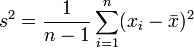## Calculate average online

How to use this average-calculator: just enter a sequence of numbers in the text-box left. You can enter a number per line (line separated), or multiple numbers on each line, separated by ; (semicolon) or a space. If your data is csv-formatted (like an export from excel or spreadsheet), you can simply copy-paste the data in the input-box below. Attn.: use . (dot) or , (comma) as decimal separator, but do not use a thousand-separator!.

Enter numbers here: cleartotal numbers 0 sum 0 average 0 variance (population) 0 standard deviation (population) 0 variance (sample) 0 standard deviation (sample) 0

## Average Calculation Formulas

#### Average (mean value)

Given a sequence of numbers, the average is calculated as (the sum of all values) divided by the number of values. (ex.: the average of 2, 3, 5, 6 and 9 is 5 as (2+3+5+6+9)/5 = 25/5 = 5 )
note: this is the unweighted average, for weighted average calculation see here.

#### Variance of a population (biased) σ2where μ is the average value of the population x1,... , xn.

#### Standard Deviation of a population (biased) σ

The standard deviation of a population σ is the square root of the variance σ2.

#### Variance of a sample (unbiased, Bessel correction factor n/(n-1))where x is the average value of the sample x1,... , xn.